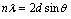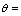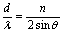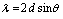1800-1023-196

+91-120-4616500

# X-Ray Study of Crystal Structure

## X-Ray Study of Crystal Structure

Study of internal structure of crystal can be done with the help of X-rays. The distance of the constituent particles can be determined from diffraction value by Bragg’s equation.Where, l = Wave length of X-rays, n = order of reflection,Angle of reflection, d = Distance between two parallel surfaces

The above equation is known as Bragg’s equation or Bragg’s law. The reflection corresponding to n = 1 (for a given family of planes) is called first order reflection; the reflection corresponding to n = 2 is the second order reflection and so on. Thus by measuring n (the order of reflection of the X-rays) and the incidence angle q, we can know d/l.From this, d can be calculated if l is known and vice versa. In X-ray reflections, n is generally set as equal to 1. Thus Bragg’s equation may alternatively be written as= 2 dhkl sinq

Where dhkl denotes the perpendicular distance between adjacent planes with the indices hkl.

## NEET & AIIMS Exam Sample Papers

 AIIMS SAMPLE PAPERS View More NEET SAMPLE PAPERS View More